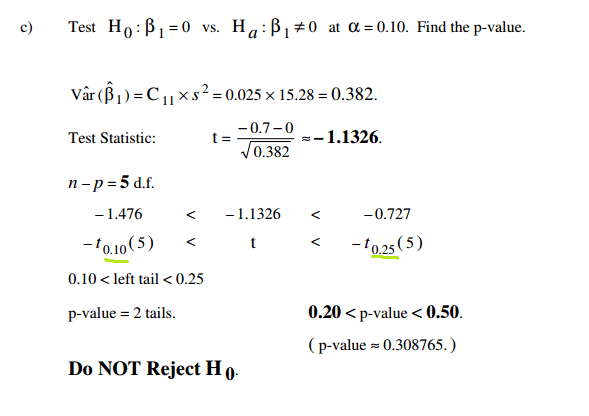# Multiple Linear Regression - Hypothesis Testing

## Homework Statement

I'm looking through some example problems that my professor posted and this bit doesn't make senseHow do you come up with the values underlined?

## The Attempt at a Solution

Upon researching it, I find that you should use α/2 for both of these values. So i'm not sure what's going on here

Homework Helper
You can't calculate the p-value exactly here, the best you can do is find a bound for it. The number of degrees of freedom is fixed at 5, so you look in the lower tail of the appropriate t-distribution, for 5 degrees of freedom, until you find two tabled values that bracket your calculated value. They just happen to be for 10% and 25%. This tells you that whatever the real p-value is, it is not smaller than 10%, so you don't reject.

$$-t_{0.025} < t < -t_{0.05}$$

you would know the p-value is smaller than 5%, so less than $\alpha = 0.05$, so you would reject.

Ray Vickson
Homework Helper
Dearly Missed
You can't calculate the p-value exactly here, the best you can do is find a bound for it. The number of degrees of freedom is fixed at 5, so you look in the lower tail of the appropriate t-distribution, for 5 degrees of freedom, until you find two tabled values that bracket your calculated value. They just happen to be for 10% and 25%. This tells you that whatever the real p-value is, it is not smaller than 10%, so you don't reject.

$$-t_{0.025} < t < -t_{0.05}$$

you would know the p-value is smaller than 5%, so less than $\alpha = 0.05$, so you would reject.

Or, you could use a package such as Maple's 'stats' facility to get a precise result:
stats[statevalf,cdf,studentst](-1.1326);
0.1543773607 <----- output
So, the p value is about 0.154.

You can't calculate the p-value exactly here, the best you can do is find a bound for it. The number of degrees of freedom is fixed at 5, so you look in the lower tail of the appropriate t-distribution, for 5 degrees of freedom, until you find two tabled values that bracket your calculated value. They just happen to be for 10% and 25%. This tells you that whatever the real p-value is, it is not smaller than 10%, so you don't reject.

$$-t_{0.025} < t < -t_{0.05}$$

you would know the p-value is smaller than 5%, so less than $\alpha = 0.05$, so you would reject.

Ok, thanks. That makes sense. I normally just try to stay away from tables and use my ti-89 instead.

For another problem, same concept:

I'm testing B3 = 0 vs b3 =/= 0 at 5% level of significance. I found a test statistic of -1.516. tistat.tcdf(-∞, -1.516, 12) = .0777. Since it's two tailed I multiply this by 2: 2(.0777) = .1554. Since .1554 > .05 I do not reject the null hypothesis. Correct? The null hypothesis is more likely than 5%

I guess I could have also done 1 - tistat.tcdf(-1.516, 1.516, 12)